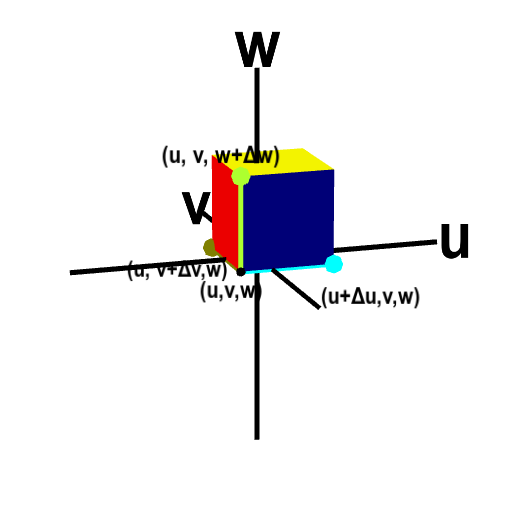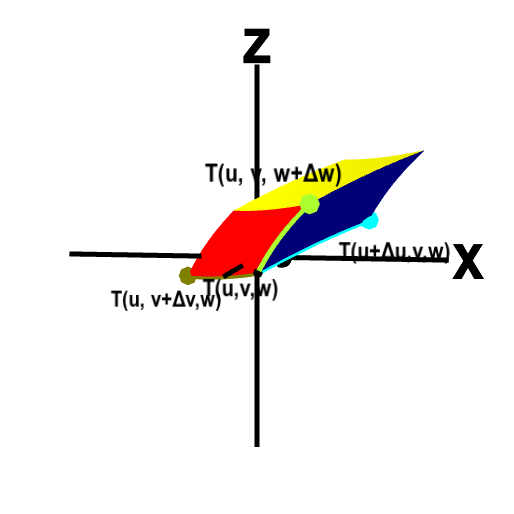# Math Insight

### Applet: Volume transformation for change of variables in triple integralsThe change of variables function $\cvarf$ transforms a small box (first panel) in $\cvarfv\cvarsv\cvartv$-space to a curvy region (second panel) in $xyz$ space, changing the volume as well as the shape. The original box has one corner at the point $(\cvarfv,\cvarsv,\cvartv)$ and its size is $\Delta\cvarfv \times \Delta\cvarsv \times \Delta\cvarfv$. The corner $(\cvarfv,\cvarsv,\cvartv)$ and the three adjacent corners are labeled. The image of those points under $\cvarf$ form adjacent corners of the curvy region. To help visualize the correspondence between the box and the curvy region under the map $\cvarf$, the edges between the labeled corners are highlighted with different colors.

You can rotate each panel with your mouse to better visual the three dimensional perspective and see all the points. You can drag any of the colored points to change the sizes $\Delta\cvarfv$, $\Delta\cvarsv$, or $\Delta\cvartv$. Dragging the box in the first panel changes the point $(\cvarfv,\cvarsv,\cvartv)$ and hence the shape of the transformation